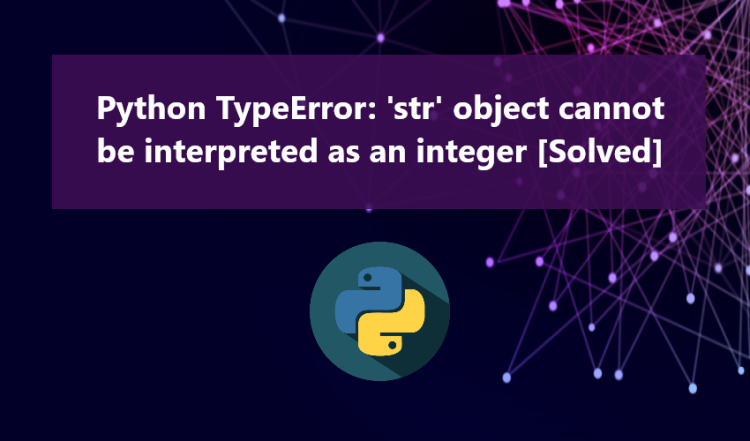# Python TypeError: 'str' object cannot be interpreted as an integer [Solved]In this article, we will explore the reasons behind and solutions for the Python TypeError, specifically when it states "TypeError: 'str' object cannot be interpreted as an integer". If you find yourself encountering this type of error during your current Python project development phase, this article aims to provide you with insights into its causes and solutions.

Python is equipped with various exceptions and errors that may arise during development, and one of these errors is the TypeError. This error can abruptly halt the execution of our script during runtime. The TypeError may manifest as follows:

Click on the Python error messages to access their respective dedicated articles for more in-depth insights.

## Why does the Python "TypeError: 'str' object cannot be interpreted as an integer" occurs?

The "TypeError: 'str' object cannot be interpreted as an integer" arises when an operation that demands an integer is attempted with a string object provided instead. This error occurs because Python expects an integer in a context where a string has been supplied, and it cannot automatically convert the string into an integer due to the conflicting data types.

For a clearer understanding, please refer to the sample Python snippet below:

`print("List All the Numbers between 2 Numbers") start = input("Enter the Starting #: ")end = input("Enter the Ending #: ") for num in range(start,end):    if start != num:        print(num)`

The above snippet is a Python script that triggers the "TypeError: 'str' object cannot be interpreted as an integer" error. This error occurs due to the use of Python's built-in function called range(). This function generates a sequence of numbers within a specific range and expects only an integer arguments, which is the root cause of the error.

## Solutions

As the range() function requires its arguments to be integers, the simple solution to resolve the exception that occurs is to convert the string object into an integer. By doing so, Python will permit the script to execute successfully. Refer to the following snippet for a clearer understanding:

`print("List All the Numbers between 2 Numbers") start = input("Enter the Starting #: ")end = input("Enter the Ending #: ") start = int(start)end = int(end) for num in range(start,end):    if start != num:        print(num)`

Furthermore, to ensure that the provided objects contain numeric values and prevent other types of errors from arising, we can add a simple conditional parameter. For this purpose, we can utilize Python's built-in method called is_numeric(). This method allows us to verify whether a string contains a numeric or non-numeric value.

Here's an example snippet that illustrates this solution:

`print("List All the Numbers between 2 Numbers") start = input("Enter the Starting #: ")end = input("Enter the Ending #: ") if start.isnumeric() == False or end.isnumeric() == False:    print("Either one or both of the provided inputs are not numeric.")else:    start = int(start)    end = int(end)     for num in range(start,end):        if start != num:            print(num)`

And if the correct type of object is provided:

## Conclusion

In simple terms, the TypeError: 'str' object cannot be interpreted as an integer occurs when an operation that requires an integer receives a string instead. By implementing the solutions provided above, you can resolve this error.

There you have it! I hope this article helps you address the error you are encountering in your Python project. Explore more on this website for more Free Source Codes, Tutorials, and Articles covering a wide range of programming languages.